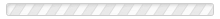• Several alternative depreciation approaches that result in relatively more depreciation in early years of use, and smaller amounts during later years
• Ordinary and necessary costs incurred to place an item of property, plant, or equipment in its condition for intended use; such amounts are included in the asset account
• A lease that effectively transfers the risks and rewards of ownership to the lessee
• A revision of assumptions used in a related accounting calculation (e.g., change in estimated useful life of an asset); handled prospectively by revising current and future periods
• An accelerated depreciation method by which a constant rate (that is a multiple of the straight-line rate) is multiplied by each period's beginning (constantly declining) book value
• Cost minus salvage value; the amount of cost that will be allocated to the service life
• An accelerated depreciation method by which a constant rate (that is 200% of the straight-line rate) is multiplied by each period's beginning (constantly declining) book value
• Includes the cost of parking lots, sidewalks, landscaping, irrigation systems, and similar expenditures that are incurred to better land
• Periodic payment from the user (lessee) of an asset to an owner (lessor) of the asset
• Periodic payment from the user (lessee) of an asset to an owner (lessor) of the asset
• Periodic payment from the user (lessee) of an asset to an owner (lessor) of the asset
• A single price paid for a package of assets; the purchase price must be allocated to each of the components
• A matter of accounting judgment; when amounts involved are slight, expediency may dictate waiving the technically correct alternative in lieu of a simpler approach
• A "depreciation" approach common to the tax code; generally permits more rapid "recovery" of asset cost than GAAP approaches; MACRS - pronounced "makers"
• A lease where the lessee makes periodic payments for periodic use of an asset, but does not assume the ultimate risks and rewards of owning the asset
• Amount expected be realized at the end of an asset's service life; "salvage value"
• The period of time that a depreciable asset will be in use by an entity; the time interval over which the asset will be depreciated
• A simple depreciation method by which the depreciable base is spread uniformly over the service life
• A depreciation approach where the depreciable base is allocated to the expected total units of output; mileage, hours, etc.